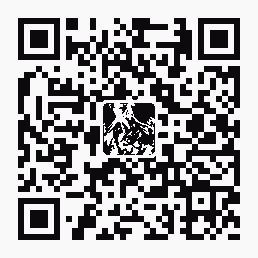| 導購 |订阅 | 在线投稿

C#系列教程 

1.隱式數值轉換

2.隱式枚舉轉換

enum Color

{

Red,Green,Blue

};

class MikeCat

{

static void Main()

{

Color c;//聲明Color的變量c;

c=0;//將0轉換爲Red;

Console.WriteLine("c的值是{0}",c);//結果：c的值是Red;如果將c=0改成c=1，則編譯器會給出錯誤。

}

} 3.隱式引用轉換

int i=(int)'A';//65 顯示轉換包含所有的隱式轉換，即任何系統允許的隱式轉換寫成顯示轉換的形式都是允許的。 int i=300;

long l=(long)i; 另外一例： using System;

class MikeCat

{

static void Main()

{

long longValue = Int64.MaxValue;

int intValue = (int) longValue;

Console.WriteLine("(int){0} = {1}", longValue, intValue);

}

} 類型 long 轉換爲 int 是顯式轉換，它使用了強制轉換表達式。輸出爲：

(int) 9223372036854775807 = -1這是因爲有溢出發生。

1.顯示數值轉換

using System;

public class MikeCat

{

public static void Main()

{

ushort u=65535;

byte b=(byte)u;

Console.WriteLine("b的值是{0}",b);

}

} 編譯狀況如下：

E:\>csc test.cs

Microsoft (R) Visual C# .NET 編譯器版本 7.10.3052.4

E:\>test.exe

b的值是255

E:\>csc/checked test.cs ///checked[+|-] 生成溢出檢查

E:\>test.exe

at MikeCat.Main()

E:\>csc/checked- test.cs

E:\>test.exe

b的值是255

2.顯示枚舉轉換

enum Color

{

Red,Green,Blue

};

public class MikeCat

{

static void Main()

{

Color c;//聲明Color的變量c;

c=(Color)4;//對數字3進行顯示枚舉轉換

Console.WriteLine("c的值是{0}",c);

}

} 結果：c的值是4

Convert 類

int i = 123; 以下語句對變量 i 隱式應用裝箱操作：

object o = i;

i 123

int i=123;

o (將i裝箱)

object o=i; int 123

int i = 123;

object o = (object) i;

// Boxing an integer variable

using System;

class TestBoxing

{

public static void Main()

{

int i = 123;

object o = i; // Implicit boxing

i = 456; // Change the contents of i

Console.WriteLine("The value-type value = {0}", i);

Console.WriteLine("The object-type value = {0}", o);

}

} 輸出

The value-type value = 456

The object-type value = 123

int i = 123; // A value type

object box = i; // Boxing

int j = (int)box; // Unboxing 下圖顯示了以上語句的結果。

i 123

int i=123;

o (將i裝箱)

object o=i; int 123

j 123

int j=(int) o;

using System;

public class UnboxingTest

{

public static void Main()

{

int intI = 123;

// Boxing

object o = intI;

// Reference to incompatible object produces InvalidCastException

try

{

int intJ = (short) o;

Console.WriteLine("Unboxing OK.");

}

catch (InvalidCastException e)

{

Console.WriteLine("{0} Error: Incorrect unboxing.",e);

}

}

} 輸出

System.InvalidCastException

at UnboxingTest.Main() Error: Incorrect unboxing.

int intJ = (short) o;

int intJ = (int) o;

󰈣󰈤

>>返回首頁<<

熱帖排行© 2005- 王朝網路 版權所有